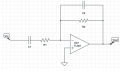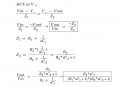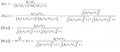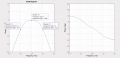# Finding R and C values for an active second-order bandpass filter

#### colinodowd

Joined Feb 22, 2023
5
I have been assigned the task to design an active bandpass filter with a passband of 0.1 to 10 Hz. At these two frequencies, there should be an attenuation of -6dB or more. So far I have selected my circuit layout which can be seen below:I found my transfer function so that I could calculate the R and C values and then converted into jw form.From here, I used math to remove the imaginary numbers and now I have my transfer function in terms of w and the components.

My questions are as follows:
1. Is the w that I need to plug in the center frequency? So sqrt(0.1*10) = 1 Hz or would it be 1*2pi?
2. Have I set up my transfer function correctly for finding when 0.1 and 10 Hz will be -6db by setting it equal to 0.5?
3. If the above are true, in theory, do I just start plugging in values for the components to make the equation equal 0.5?#### crutschow

Joined Mar 14, 2008
32,064
Is that the only requirements for the filter?

That circuit is just effectively two 1st order, passive RC filters in series with an op amp buffer, and has the low-Q, slow rolloff characteristics of such filters.
For an active filter with a sharper rolloff, look up Sallen-Key or Multiple-Feedback 2nd order designs.

#### colinodowd

Joined Feb 22, 2023
5
Is that the only requirements for the filter?

That circuit is just effectively two 1st order, passive RC filters in series with an op amp buffer, and has the low-Q, slow rolloff characteristics of such filters.
For an active filter with a sharper rolloff, look up Sallen-Key or Multiple-Feedback 2nd order designs.
Yes, these are the only requirements. I have found R and C values that get me the rolloff that I need but I don't know how to prove it mathematically. I don't know how I need to manipulate my transfer function to find these values.

R1=R2=100k
C1 = 9.2uF
C2 = 275nF

These numbers plugged into my transfer function get me the attached output:

clear all;
s = tf('s');
G = (0.92*s)/((0.92*s+1)*(0.0275*s+1));

figure(1)
opts1=bodeoptions('cstprefs');
opts1.PhaseVisible = 'off';
opts1.YLim={[-20 10]};
opts1.FreqUnits = 'Hz'; % or 'rad/second', 'rpm', etc.
Mag=subplot(1,2,1);bodeplot(G,opts1); grid on;
opts2=bodeoptions('cstprefs');
opts2.MagVisible = 'off';
opts2.YLim={[-180 180]};
opts2.FreqUnits = 'Hz'; % or 'rad/second', 'rpm', etc.
Phase=subplot(1,2,2);bodeplot(G,opts2); grid on; title('');#### crutschow

Joined Mar 14, 2008
32,064
these are the only requirements.
Okay.
I wasn't sure since your thread title says "second-order bandpass filter" and it is not that.

•MrAl

#### colinodowd

Joined Feb 22, 2023
5
Okay.
I wasn't sure since your thread title says "second-order bandpass filter" and it is not that.
Oh I figured it was second order because of the two active components and the transfer function has two poles.

#### Papabravo

Joined Feb 24, 2006
20,153
Oh I figured it was second order because of the two active components and the transfer function has two poles.
Cascading two 1st order filters is not the same thing as having a second order filter. You can tell the difference in two different ways when the transfer function has two poles..
1. If the slope of the rolloff is 20 dB/decade, you have multiple first order responses.
2. If both poles of the transfer function are on the negative real axis you have cascaded 1st order sections. If the poles form a complex conjugate pair you have a 2nd order response.
Filters with an odd order usually have a single 1st order section followed by some number of 2nd order sections, each of which has a different Q, because each complex conjugate pair has a different characteristic angle with the negative real axis.

#### colinodowd

Joined Feb 22, 2023
5
Cascading two 1st order filters is not the same thing as having a second order filter. You can tell the difference in two different ways when the transfer function has two poles..
1. If the slope of the rolloff is 20 dB/decade, you have multiple first order responses.
2. If both poles of the transfer function are on the negative real axis you have cascaded 1st order sections. If the poles form a complex conjugate pair you have a 2nd order response.
Filters with an odd order usually have a single 1st order section followed by some number of 2nd order sections, each of which has a different Q, because each complex conjugate pair has a different characteristic angle with the negative real axis.
Okay, got it. I was going based off the definition of the filter I am using from this website. https://www.electrical4u.com/band-pass-filter/

#### BobaMosfet

Joined Jul 1, 2009
2,073
You may find this book useful now, or in the future--

Active Filter Cookbook; 2nd Ed.
Author: Don Lancaster
ISBN-13: 978-0750629867
ISBN-10: 075062986X

#### colinodowd

Joined Feb 22, 2023
5
Bumping to see if I can get any help on the problem.

I did make some more progress on how to solve for the values of the high pass filter. Still stuck on the low pass though.#### The Electrician

Joined Oct 9, 2007
2,927
Your result is very close to the exact result.

To get the exact result you must solve two simultaneous equations for C1 and C2. You need to have an expression for the magnitude of your transfer equation and set it equal to 1/2 at the two cutoff frequencies (.1 and 10). This will give you the two equations.

One problem with doing this is that your expression for |H(jw)| in post #1 is incorrect. You'll need to research the correct way to form that expression. Hint: it involves the complex conjugate.

Edit: I see that the only thing that makes your final expression for |H(jw)| incorrect is that in the numerator of that expression you have R1 where you should have R2.

Last edited:

#### The Electrician

Joined Oct 9, 2007
2,927
colinodowd, have you had any luck deriving the correct expression for |H(jw)|?

#### Audioguru again

Joined Oct 21, 2019
5,820
Here is my simulation of your simple bandpass filter:

#### MrAl

Joined Jun 17, 2014
10,087
Hello there,

There is a slight discrepancy about what a second order response is in this thread.
A second order response is a response that does not reduce to a first order response, usually meaning that the denominator has an 's' power of 2 in the denominator when expanded, and there is no numerator factor that can cancel one of the denominator factors. A first order response, when reduced as much as possible, only has a power of 1 for 's' in the denominator.

The correct terminology for second order responses is either over damped, under damped, or critically damped, but even the over damped (like this thread has) is still a second order response.

Another way of looking at it is that a second order response has the ability to change twice, while a first order only once, so a first order response either goes up all the time or down all the time, and possibly they can flatten out, but a second order response can go up for a while then come back down, or go down for a while then go back up.
So in general there will be 2 changes for a second order response and only one for a first order response, although the changes may be subtle.

A true Bode plot for a first order low pass and first order high pass will show how this works better.